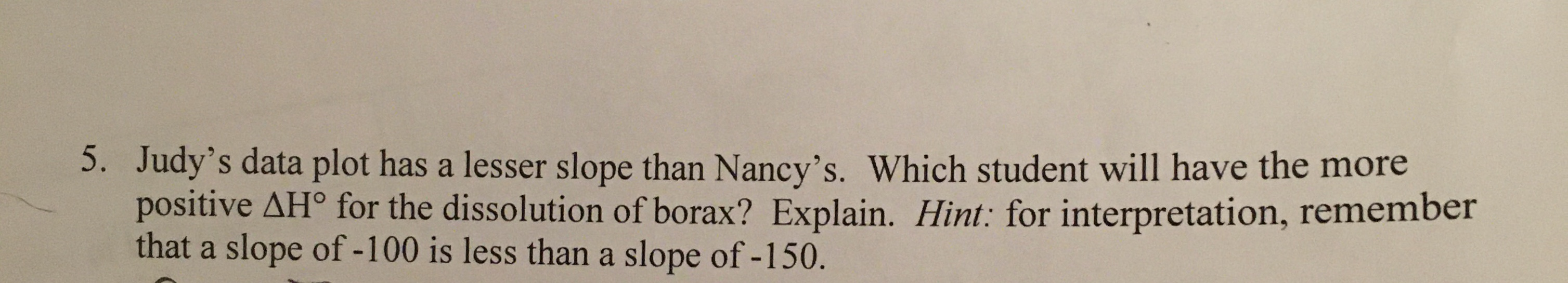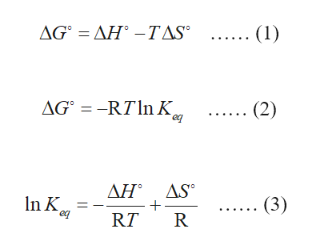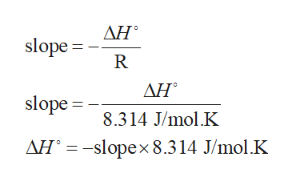# 5. Judy's data plot has a lesser slope than Nancy's. Which student will have the morepositive AH° for the dissolution of borax? Explain. Hint: for interpretation, rememberthat a slope of -100 is less than a slope of -150.

Question
16 viewshelp_outlineImage Transcriptionclose5. Judy's data plot has a lesser slope than Nancy's. Which student will have the more positive AH° for the dissolution of borax? Explain. Hint: for interpretation, remember that a slope of -100 is less than a slope of -150. fullscreen
check_circle

Step 1

The equation for Gibbs free energy is written in equation (1).

The Gibbs free energy in equilibria is written in equation (2).

Combine equations (1) and (2), as shown in equation (3). This equation is in the form of straight line represented by y = mx + b.help_outlineImage TranscriptioncloseΔGΔΗ -TAS (1) ΔG-RT In K, (2) eg ΔΗ ΔS. In K (3) RT R Θ fullscreen
Step 2

The straight line with a slope equal to -∆H/R is formed in t...help_outlineImage TranscriptioncloseΔΗ. slope = R AH ΔΗ' slope = 8.314 J/mol.K AH° =-slope× 8.314 J/mol.K fullscreen

### Want to see the full answer?

See Solution

#### Want to see this answer and more?

Solutions are written by subject experts who are available 24/7. Questions are typically answered within 1 hour.*

See Solution
*Response times may vary by subject and question.
Tagged in

### Chemical Thermodynamics